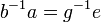# Sufficiency of subgroup criterion

## Contents

This article gives the statement, and possibly proof, of a basic fact in group theory.
View a complete list of basic facts in group theory
VIEW FACTS USING THIS: directly | directly or indirectly, upto two steps | directly or indirectly, upto three steps|
This article gives a proof/explanation of the equivalence of multiple definitions for the term subgroup
View a complete list of pages giving proofs of equivalence of definitions

## Statement

For a subset$H$ of a group$G$, the following are equivalent:

1.$H$ is a subgroup, viz$H$ is closed under the binary operation of multiplication, the inverse map, and contains the identity element
2.$H$ is a nonempty set closed under left quotient of elements (that is, for any$a, b$ in$H$,$b^{-1}a$ is also in$H$)
3.$H$ is a nonempty set closed under right quotient of elements (that is, for any$a, b$ in$H$,$ab^{-1}$ is also in$H$)

## Proof

We shall here prove the equivalence of the first two conditions. Equivalence of the first and third conditions follows by analogous reasoning.

### (1) implies (2)

Clearly, if$H$ is a subgroup:

•$H$ is nonempty since$H$ contains the identity element
• Whenever$a, b$ are in$H$ so is$b^{-1}$ and hence$b^{-1}a$

### (2) implies (1)

Suppose$H$ is a nonempty subset closed under left quotient of elements. Then, pick an element$u$ from$H$. (VIDEO WARNING: In the embeddded video, the letter$a$ is used in place of$u$, which is a little unwise, but the spirit of reasoning is the same).

•$e$ is in$H$: Set$a = b = u$ to get$u^{-1}u$ is contained in$H$, hence$e$ is in$H$
•$g \in H \implies g^{-1} \in H$: Now that$e$ is in$H$, set$b = g, a =e$ to get$b^{-1}a = g^{-1}e$ is also in$H$, so$g^{-1}$ is in$H$
•$x,y \in H \implies xy \in H$: Set$a = y, b= x^{-1}$. The previous step tells us both are in$H$. So$b^{-1}a = (x^{-1})^{-1}y$ is in$H$, which tells us that$xy$ is in$H$.

Thus,$H$ satisfies all the three conditions to be a subgroup.